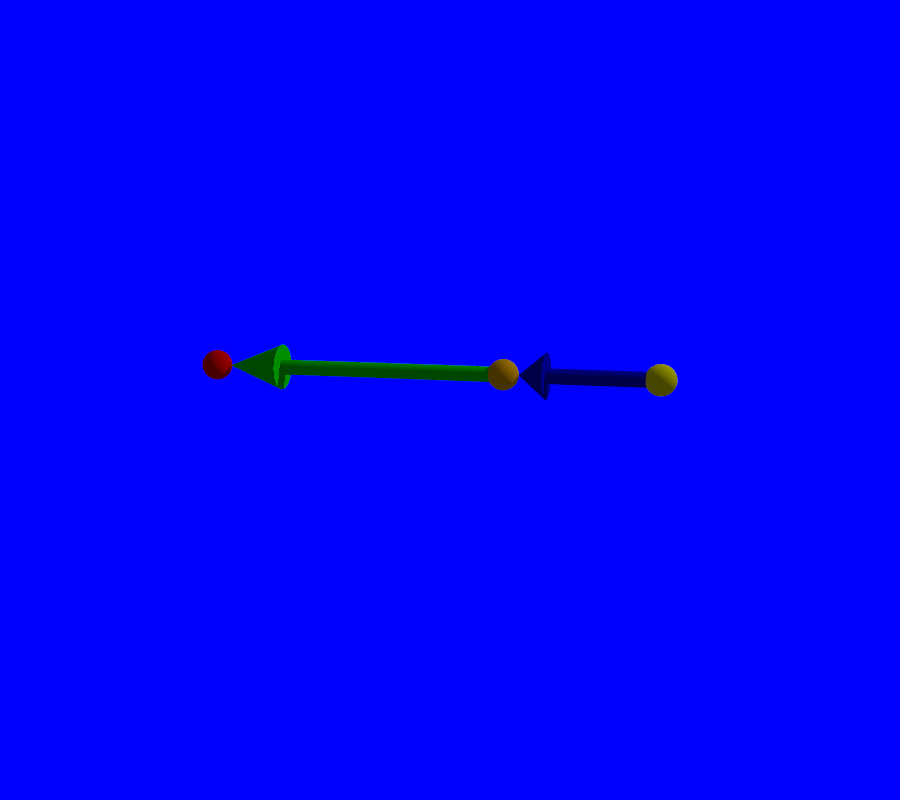# Points, vectors and barycenters

## Points and Vectors

In the preceding chapters, we have used points and vectors. Here we recall the basics notions of points, vectors, and barycenters for those who have forgotten math. Then we show how they are implemented in Pycao.

Usually, points and vectors are displayed picturally as follows.Recall that we have the formulas :

\begin{align}\begin{aligned}point+vector=point\\point-point=vector\\vector+vector=vector\end{aligned}\end{align}

In the above picture, we have:

\begin{align}\begin{aligned}yellow point+blueVector=orangePoint\\redPoint-orangePoint=GreenVector\\blueVector+greenVector=redPoint-yellowPoint\end{aligned}\end{align}

This formalism is known to pycao. Let us see the implementation of the above picture as an illustration, and let’s check the above formulas.

yellowPoint=point(1,2,3)
blueVector=vector(1,0,0)
greenVector=vector(2,0,0)
orangePoint=yellowPoint+blueVector
redPoint=greenVector+orangePoint

print("Blue+Green")
print(blueVector+greenVector)
print("Red-Yellow")
print(redPoint-yellowPoint)
print("Red")
print(redPoint)
print("Yellow")
print(yellowPoint)

def gVector(start,end,cylinderRadius,arrowPercentage,arrowRadius,color):
#print(start)
start=start.copy()
#print(start)
end=end.copy()
endCylinder=(1-arrowPercentage)*start+arrowPercentage*end
basket=ObjectInWorld()
basket=point(0,0,0)
cyl=Cylinder(start,endCylinder,cylinderRadius).colored(color).glued_on(basket)
cone=Cone(endCylinder,end,arrowRadius,0).colored(color).glued_on(basket)
#print(cone)
return basket

yp=Sphere(yellowPoint,0.1).colored("Yellow")
op=Sphere(orangePoint,0.1).colored("Orange")
rp=Sphere(redPoint,0.1).colored("Red")
bv=gVector(yellowPoint,orangePoint-.1*X,0.05,0.8,0.15,"Blue")
gv=gVector(orangePoint,redPoint-.1*X,0.05,0.8,0.15,"Green")
gv.name="greenVector"

camera=Camera()
camera.file="pointsAndVectors.pov"
camera.location=origin-0*X-6*Y+4*Z
#camera.lights=[light]
camera.actors=[yp,op,rp,bv,gv] # what is seen by the camera
camera.lookAt=.5*(yp.center+rp.center)
camera.zoom(.8)
camera.filmAllActors=True
#camera.actors=[gv.children]
#camera.actors=[grayGround,brownCube,closedPinkCylinder,infiniteYellowCylinder,violetCone,
#            redSphere,silverWasher,blueOpenCylinder,yellowTorus,cyanLathe,orangeLathe,bronzeLathe,HunterGreenRuledSurface]
camera.shoot # takes the photo, ie. creates the povray file, and stores it in camera.file
camera.show # show the photo, ie calls povray.


The print section of the code gives the expected results

Blue+Green
Vector  [3 0 0]
Red-Yellow
Vector  [3 0 0]
Red
Affine Point  [4 2 3]
Yellow
Affine Point  [1 2 3]


Recall that it is not allowed to add 2 points : this makes no sense. For instance

print(yellowPoint+redPoint)
>>Mass Point  [5 4 6 2]


In pycao, the output is a massic point of mass 2 (the last coordinate), neither a point nor a vector. A vector is a massic point of mass 0 and a usual point a massic point of mass 1.

On the other hand, it makes sense to take linear combinations of points as long as the sum of coefficients is 1: this is a barycenter. For instance, the point center of two points p1 and p2 is defined as a barycenter by the formula:

center=0.5*p1+0.5*p2


In general, besides the center, you can use barycenters with pycao with formulas:

point=n1*p1+n2*p2+...+nk*pk


if n1+n2+...nk==1

## Aliases

We have aliases to speed up the input and for readability.

X=vector(1,0,0)
Y=vector(0,1,0)
Z=vector(0,0,1)
T=point(0,0,0)
origin=point(0,0,0)
plane=AffinePlaneWithEquation
line=Segment


In principle, the constructors of objects start with a capital letter (MassPoint,Cone,Cylinder,Cube...). This is the standard convention for Python classes. However, for the objects we use so much, we have inserted alias with or without capital letter so that both are usable (origin or Origin,point or Point,vector or Vector,line or Line,plane or Plane).

## Maps and vectors

When an affine map is applied to a vector, it is the underlying linear map which is applied, as this is the operation which makes sense mathematically. For instance, a vector remains unchanged if a translation is applied (Intuitivly, the two extremal points of the vectors are moved, thus the vector joining these two points remains unchanged).